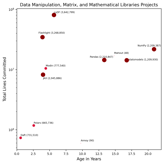# Data Manipulation, Matrix, and Mathematical Libraries¶

Description Libraries that provide data manipulation and mathematical primitives. Many of these libraries are key components used by other data science projects. 11## Projects¶

Project

Size Score

Trend Score

Byline

Annoy

3.25

2.75

Approximate Nearest Neighbors in C++/Python optimized for memory usage and loading/saving to disk

cuDF

7.0

6.0

GPU dataframe library

Daft

2.5

7.0

The Python DataFrame for Complex Data

Flashlight

7.5

6.5

A C++ standalone library for machine learning

JAX

6.25

6.5

Composable transformations of Python+NumPy programs: differentiate, vectorize, JIT to GPU/TPU, and more

Mahout

4.75

2.25

Apache Mahout is a distributed linear algebra framework and mathematically expressive Scala DSL designed to let mathematicians, statisticians, and data scientists quickly implement their own algorithms.

Modin

5.0

7.5

Speed up your Pandas workflows by changing a single line of code

NumPy

8.25

4.0

The fundamental package for scientific computing with Python.

Pandas

7.25

5.25

Flexible and powerful data analysis / manipulation library for Python, providing labeled data structures similar to R data.frame objects, statistical functions, and much more.

Polars

4.0

9.75

Fast multi-threaded, hybrid-streaming DataFrame library in Rust | Python | Node.js

statsmodels

7.25

5.75

Statistical modeling and econometrics in Python